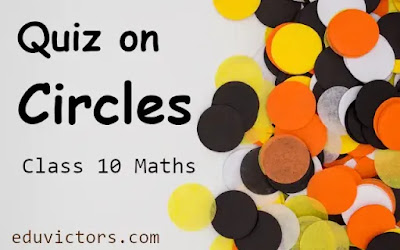# Quiz on Circles -  Class 10 Mathematics

Q1: A line intersecting a circle in two points is called a ___.

(a) tangent

(b) secant

(c) diameter

(d) sector

Q2: At most, for a given tangent a circle can have how many parallel tangents

(a) 0

(b) infinite

(c) 2

(d) 3

Q3: The angle between the radius and the tangent at the point of contact is

(a) 0°

(b) 45°

(c) 60°

(d) 90°

Q4: A tangent PQ at a point P of a circle of radius 7 cm meets a line through thecentre O at point Q so that OQ = 25 cm. Length PQ is ____.

(a) 24 cm

(b) 7cm

(c) 5 cm

(d) √(119)

Q5: If the angle between two radii of a circle is 110º, then the angle between the tangents at the ends of the radii is:

(a) 70º

(b) 40º

(c) 50º

(d) 90º

Q6: AB is a chord of the circle and AOC is its diameter such that ∠ACB = 50°. If AT is the tangent to the circle at the point A, then BAT is equal to

(a) 40°

(b) 50°

(c) 60°

(d) 65°

Q7: If a parallelogram circumscribes a circle, then it is a:

(a) Rhombus

(b) Rectangle

(c) Square

(d) None of these

Q8: The number of tangents drawn at an interior point of the circle is/are:

(a) Infinite

(b) None

(c) One

(d) Two

Q9: The tangents drawn at the extremities of the diameter of a circle are:

(a) equal

(b) perpendicular

(c) parallel

(d) none of these

Q10: The length of a tangent from an external point X on a circle with centre O is always less than OX

(a) False

(b) True

(c) Either True or False

(d) None of these

1: (b) secant

2: (c) 2

3: (d) 90°

4: (a) 24 cm

5: (a) 70º

6: (b) 50°

7: (a) Rhombus

8: (b) None

9: (c) parallel

10: (b) True